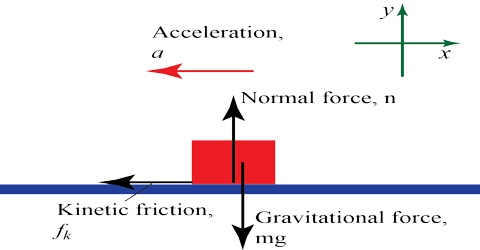# Nature of Gravitational Force

Nature of Gravitational Force:

A force is a push or pulls acting upon an object as a result of its interaction with another object. Among all the forces of Nature, the gravitational force is the one that has been notorious to man for the highest time. One of its fundamental properties -that all bodies fall with the same acceleration- was recognized by Galileo at the beginning of the seventeenth century.

Gravitational force is the mutual attractive force between two bodies. Two changed bodies or two magnets attract each other when the charge is inversely related i.e., one is positive and the other is negative and repulses when the two charges are homogeneous or of same property. Gravitational force is the weakest force in nature. In case of magnet there is attraction when the opposite poles/ends of the two magnets come close to each other and repulses when the two poles/ends are homogeneous or of same property. But gravitational is only attractive force. Gravitational force acts irking the pining straight line of the two bodies. Besides, gravitational force does not depend on medium. Whatever may be the media, there is no change of this force. This force does not change whatever the medium is.

Gravitational force properties:

• Gravitational force is always attractive. For example, earth always attracts us but never repels.
• It is weakest force in nature.
• Gravitational force is a central force.
• It is a conservative force.
• They can operate over a very long distances.
• Graviton is the particle exchanged when the two masses attract through gravitational force. This exchanged particle is called graviton.